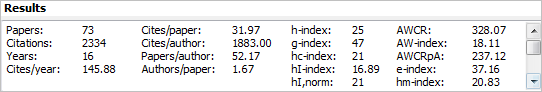# 2.2 Citation metrics

Publish or Perish calculates the following citation metrics.

• Total number of papers
• Total number of citations
• Years active
• Average number of citations per year
• Average number of citations per paper
• Average number of citations per author
• Average number of papers per author
• Average number of authors per paper
• Hirsch's h-index and related parameters, shown as h-index and Hirsch a=y.yy, m=z.zz in the output. [a and m are shown below the fold]
• Egghe's g-index, shown as g-index in the output
• The contemporary h-index, shown as hc-index and ac=y.yy in the output
• Three variations of the individual h-index, shown as hI-index, hI,norm, and hm-index in the output
• The age-weighted citation rate and related metrics
• Zhang's e-index.

Calculation of these metrics is explained below roughly in the order in which they appear in the screenshot below. Several less frequently used metrics, such as Hirsch's a and m, are shown “below the fold” and can be accessed by using the scroll bar on the right.The various basic statistics (number of papers, number of citations, citations per paper/year and citations/papers per author) in the two most left-hand columns are mostly self-explanatory and will be discussed only very briefly below.

I also provide a brief introduction of the more complex metrics. For a more extensive explanation of these metrics as well as an evaluation of their advantages and disadvantages, see Section 1.4 Citation Metrics.